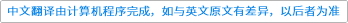Journal of the ACM ( IF 1.686 ) Pub Date : 2021-01-13 , DOI: 10.1145/3431922
Iddo Tzameret; Stephen A. Cook

Aiming to provide weak as possible axiomatic assumptions in which one can develop basic linear algebra, we give a uniform and integral version of the short propositional proofs for the determinant identities demonstrated over GF(2) in Hrubeš-Tzameret . Specifically, we show that the multiplicativity of the determinant function and the Cayley-Hamilton theorem over the integers are provable in the bounded arithmetic theory VNC2; the latter is a first-order theory corresponding to the complexity class NC2 consisting of problems solvable by uniform families of polynomial-size circuits and O(log2 n)-depth. This also establishes the existence of uniform polynomial-size propositional proofs operating with NC2-circuits of the basic determinant identities over the integers (previous propositional proofs hold only over the two-element field).down
wechat
bug